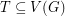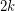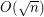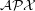# Disjoint paths

## Kriesell's Conjecture ★★

Author(s): Kriesell

Conjecture   Letbe a graph and letsuch that for any pairthere areedge-disjoint paths fromtoin. Thencontainsedge-disjoint trees, each of which contains.

## Approximation Ratio for Maximum Edge Disjoint Paths problem ★★

Author(s): Bentz

Conjecture   Can the approximation ratiobe improved for the Maximum Edge Disjoint Paths problem (MaxEDP) in planar graphs or can an inapproximability result stronger than-hardness?